Interval and segment

(diff) ← Older revision | Latest revision (diff) | Newer revision → (diff)

The simplest sets of points on the line. An interval (open interval) is a set of points on a line lying between two fixed pointsand, whereandthemselves are considered not to belong to the interval. A segment (closed interval) is a set of points between two pointsand, whereandare included. The terms "interval" and "segment" are also used for the corresponding sets of real numbers: an interval consists of numberssatisfying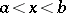, while a segment consists of thosefor which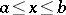. An interval is denoted by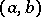, or, and a segment by.

The term "interval" is also used in a wider sense for arbitrary connected sets on the line. In this case one considers not onlybut also the infinite, or improper, intervals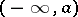,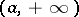,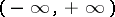, the segment, and the half-open intervals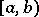,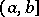,,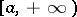. Here, a round bracket indicates that the appropriate end of the interval is not included, while a square bracket indicates that the end is included.

BSE-3

The notion of an interval in a partially ordered set is more general. An intervalconsists in this setting of all elementsof the partially ordered set that satisfy. An interval in a partially ordered set that consists of precisely two elements is called a simple or an elementary interval.

L.A. Skornyakov